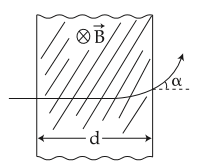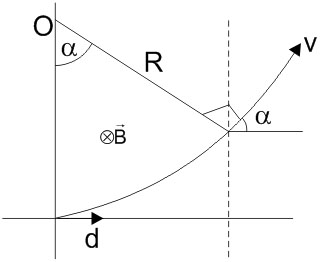#### A proton (mass m) accelerated by a potential difference V flies through a uniform transverse magnetic field B.  The field occupies a region of space by width 'd'. If 'a' be the angle of deviation of proton from initial direction of motion (see figure), the value ofwill  be :Option 1)Option 2)Option 3)Option 4)As we learnt in-Energy of ProtonMagnetic forceFrom the figureOption 1)Incorrect

Option 2)Incorrect

Option 3)Correct

Option 4)Incorrect

##### Posted by